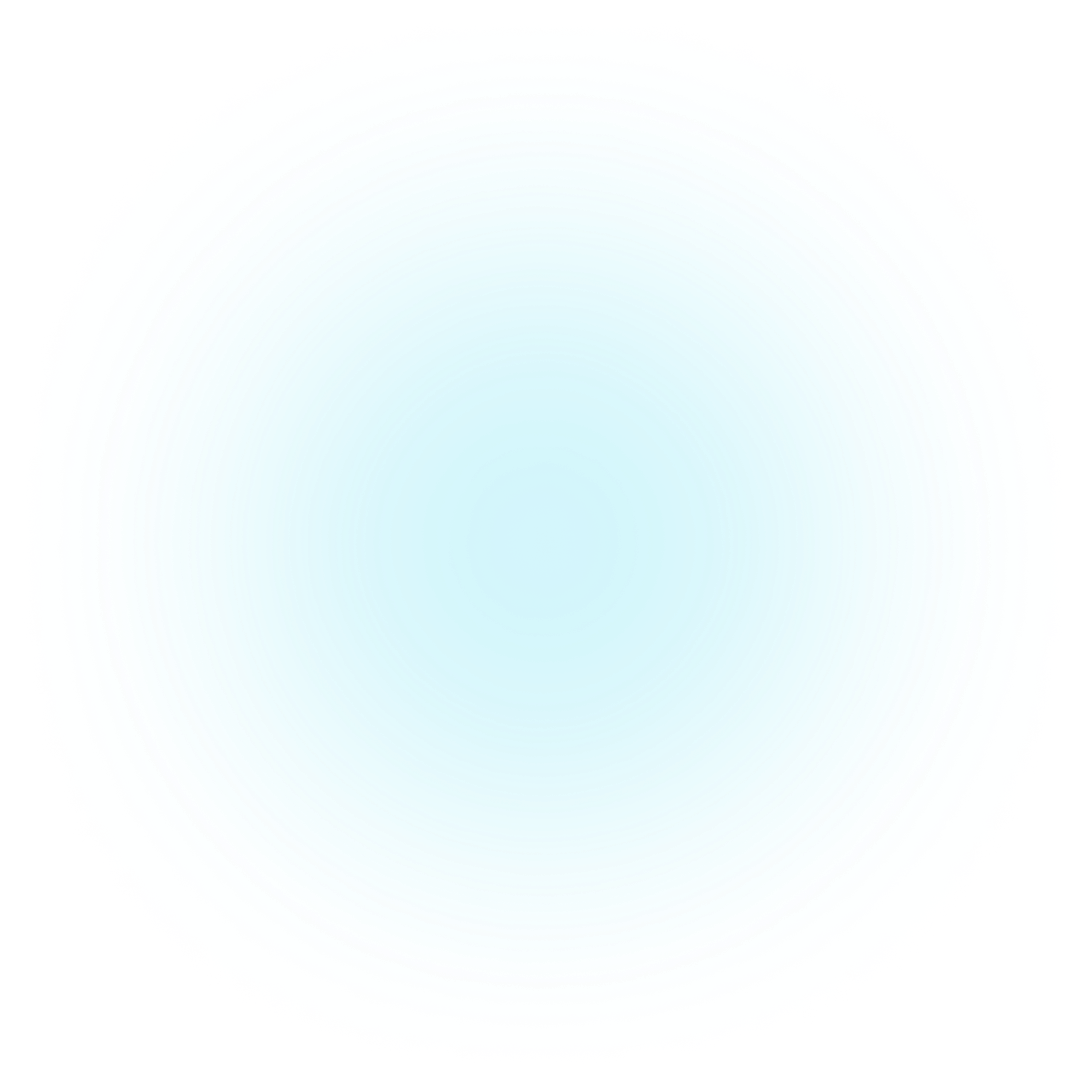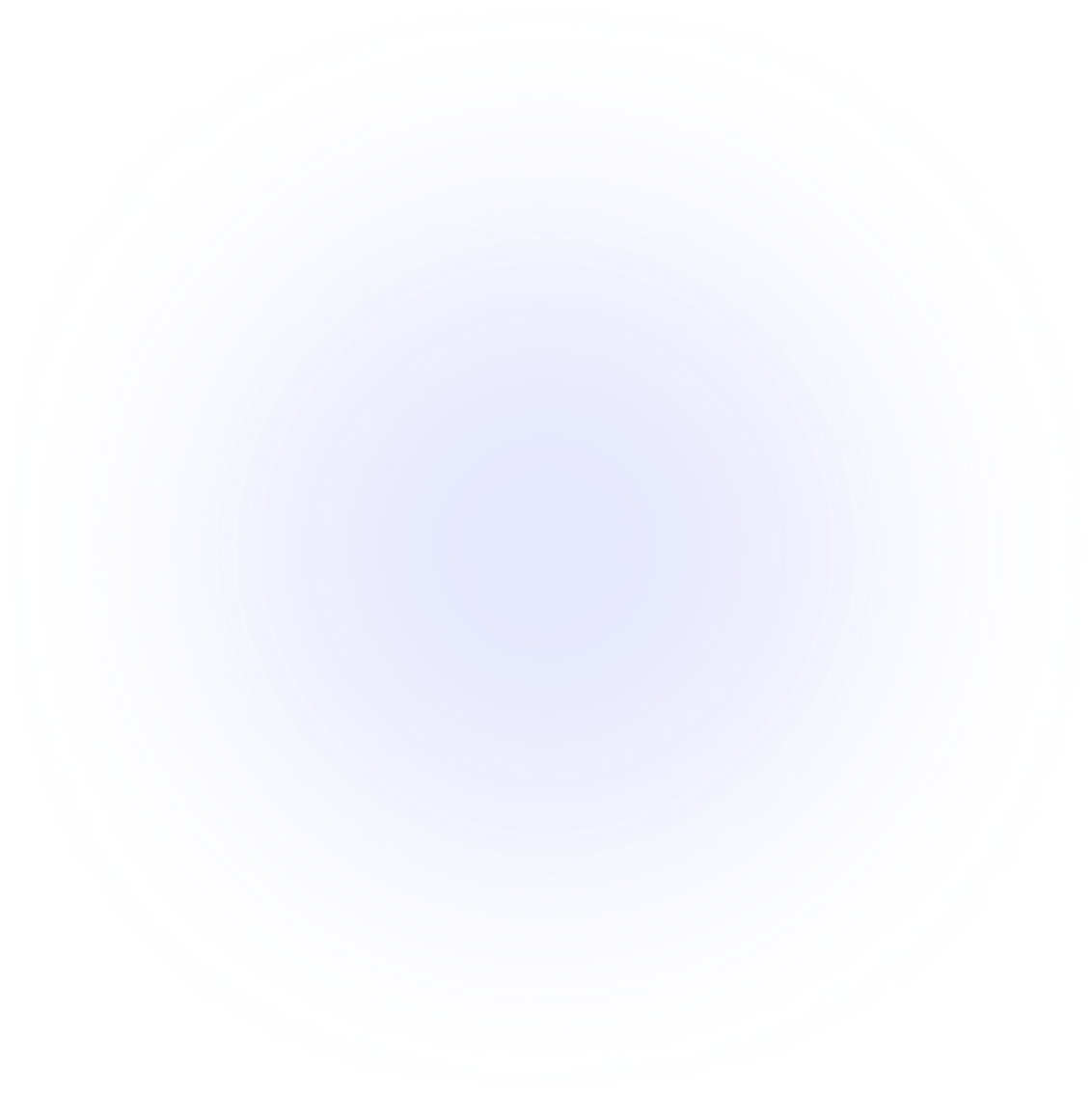Easily turn your data into engaging visualizations. Powerful API for coders. Powerful app for everyone.main.py
notebook.ipynb
main.rs
from plotapi import Chord

Chord(matrix, names).show()

Visualizations Terminus

# Pipe styling

The Terminus pipes can be modified to improve the presentation of the diagram, giving control over the spacing and slope positions.

## Sample data

Let's import PlotAPI and load our sample data.

from plotapi import Terminus

{"source":"England", "target":"Germany", "value": 1000},
{"source":"England", "target":"France", "value": 3000},
{"source":"England", "target":"Spain", "value": 5000},
{"source":"England", "target":"Italy", "value": 4000},
{"source":"England", "target":"Japan", "value": 800},

{"source":"Ireland", "target":"Germany", "value": 3500},
{"source":"Ireland", "target":"France", "value": 3750},
{"source":"Ireland", "target":"Spain", "value": 1750},
{"source":"Ireland", "target":"Italy", "value": 5000},
{"source":"Ireland", "target":"Japan", "value": 400},
]


## Demonstration

There are many opportunities to customise the Terminus pipes.

• slope_start, to change the point at which the pipe starts transitioning from its source $x$ (or $y$) position to its target $x$ (or $y$) position. Whether it's $x$ or $y$ depends on whether it's a vertical Terminus diagram or not. This value must be a float between or equal to $0.0$ and $1.0$.
• slope_end, similar to the above, but for when the sope ends.
• target_spacing_ratio, to specify how much of the arrangement should be whitespace versus how much should be taken up by pipe thickness. This value must be a float between or equal to $0.0$ and $1.0$.

Let's try a few combinations.

Terminus(links, pipe_color="#000000", pipe_opacity=0.1, show_stats=False,
slope_start=0.1, slope_end=0.9).show()

Terminus(links, pipe_color="#000000", pipe_opacity=0.1, show_stats=False,
slope_start=0.45, slope_end=0.55).show_png()Terminus(links, pipe_color="#000000", pipe_opacity=0.1, show_stats=False,
pipe_spacing_ratio=0.8).show_png()Previous
Terminus